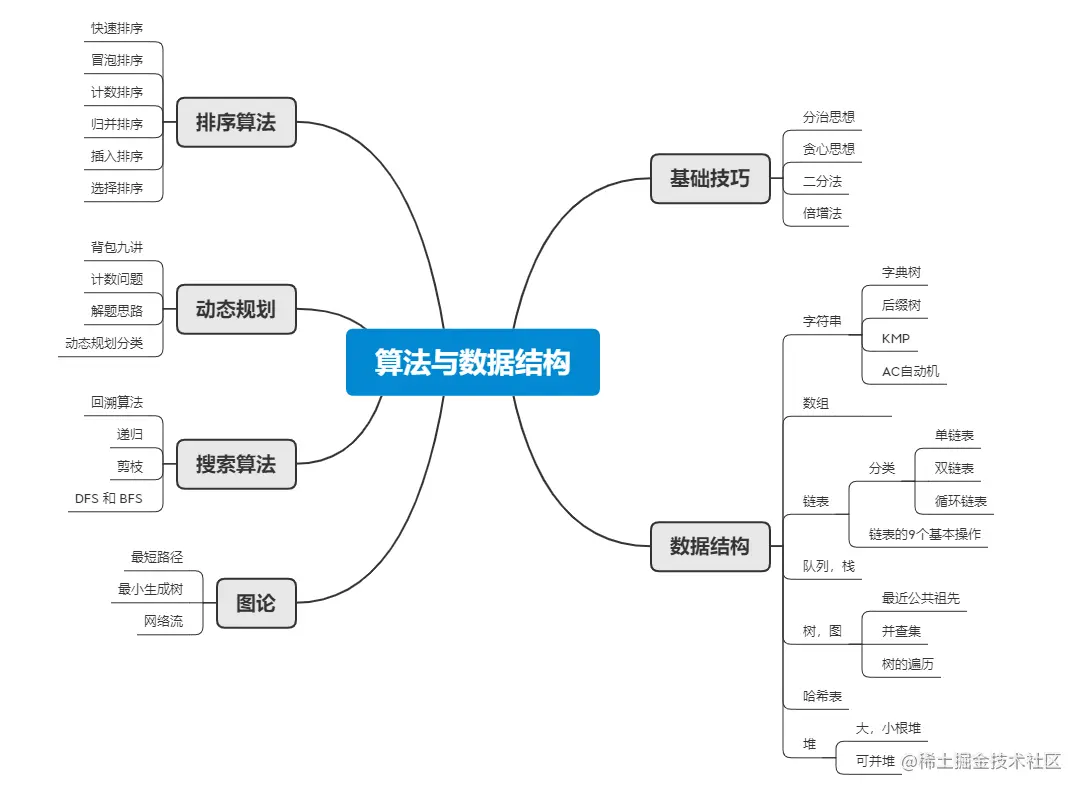# 「算法与数据结构」你可能需要的一份前端算法总结

56643

## 前言• 常用数据结构

• 数组，字符串
• 链表
• 队列
• 高级数据结构

• 前缀树
• 线段树
• 树状数组
• 主席树

## 排序算法

``````const merge = (left, right) => { // 合并数组

let result = []
// 使用shift()方法偷个懒,删除第一个元素,并且返回该值
while (left.length && right.length) {
if (left <= right) {
result.push(left.shift())
} else {
result.push(right.shift())
}
}
while (left.length) {
result.push(left.shift())
}

while (right.length) {
result.push(right.shift())
}
return result
}

let mergeSort = function (arr) {
if (arr.length <= 1)
return arr
let mid = Math.floor(arr.length / 2)
// 拆分数组
let left = arr.slice(0, mid),
right = arr.slice(mid);
let mergeLeftArray = mergeSort(left),
mergeRightArray = mergeSort(right)
return merge(mergeLeftArray, mergeRightArray)
}

// let arr = [2, 9, 6, 7, 4, 3, 1, 7, 0, -1, -2]
// console.log(mergeSort(arr))
``````

## 动态规划

• 概念
• 动态规划解决了什么问题
• 动态规划解题的步骤
• 如何高效率刷dp专题

Wikipedia 定义：它既是一种数学优化的方法，同时也是编程的方法。

1. 状态定义
2. 列出状态转移方程
3. 初始化状态

「算法与数据结构」一张脑图带你看动态规划算法之美强烈推荐这篇问题，里面讲的很清楚了。

• 首先，我先看题解，把它的状态转移方程写下来，仔细的品味一下，它这么定义，解决了我之前的什么难点，为啥我是没有想到的。
• 然后，看完之后，尝试按照这个题解思路，我自己能不能单独实现呢？
• 如果不能的话，就照着它的代码，写一遍，多看看状态转移方程是如何写的，把这个题目收藏起来。
• 等到下次，或者是隔天，再来看一遍题目，然后看看能不能单独完成，如果不能，第三天再这么操作。

## ❤️ 感谢大家

1. 点赞支持下吧，让更多的人也能看到这篇内容（收藏不点赞，都是耍流氓 -_-）
2. 关注公众号前端UpUp，联系作者👉 DayDay2021 ，我们一起学习一起进步。
3. 觉得不错的话，也可以阅读TianTian近期梳理的文章（感谢掘友的鼓励与支持🌹🌹🌹）：
•••••••••••